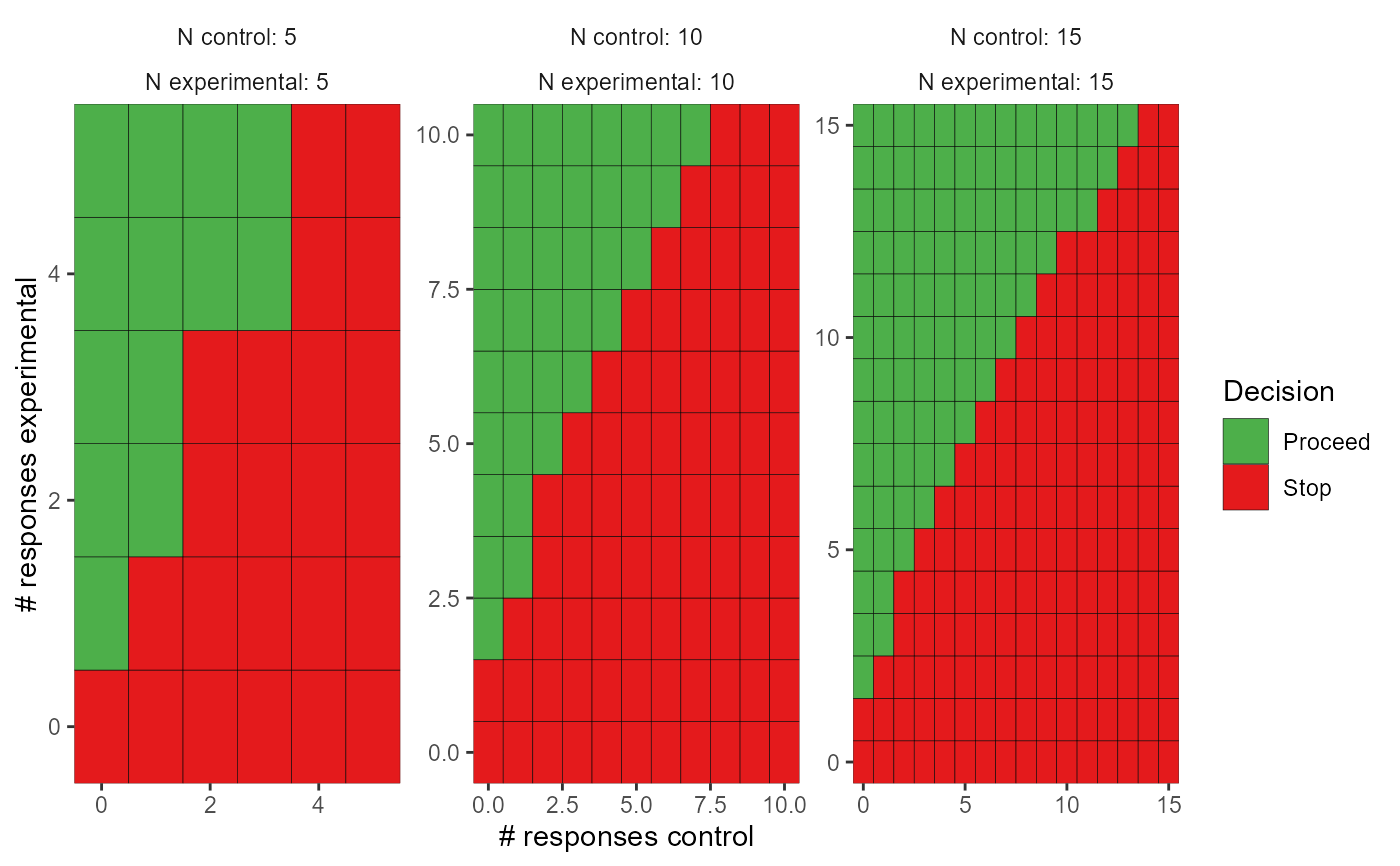Returns a plot of decision rules from the results of calc_decision_rules that can interactively show when to stop and when to proceed at the various interim analyses

## Usage

# S3 method for calc_decision_rules
plot(x, plotly = TRUE, ...)

## Arguments

x

an object of class 'calc_decision_rules', usually returned by the calc_decision_rules function

plotly

should the plot be rendered in plotly? (Default is TRUE)

...

unused

## Value

In the one-sample case, a heatmap plot with number enrolled on the x-axis and number of responses on the y-axis. In the two-sample case, a grid of heatmap plots. Each plot is a combination of the number enrolled so far in the experimental and control arms. The x-axis is the number of responses in the control arm and the y-axis is the number of responses in the experimental arm. Green indicates combinations where the trial would proceed and red indicates combinations where the trial would stop.

## Examples


set.seed(123)

# Two-sample case
dec_tbl <- calc_decision_rules(
n = cbind(seq(5, 15, 5), seq(5, 15, 5)),
N = c(15, 15),
theta = 0.86,
ppp = 0.2,
p0 = NULL,
direction = "greater",
delta = 0,
S = 50
)

plot(dec_tbl, plotly = FALSE)
#> Joining, by = c("n0", "n1", "r0", "r1")